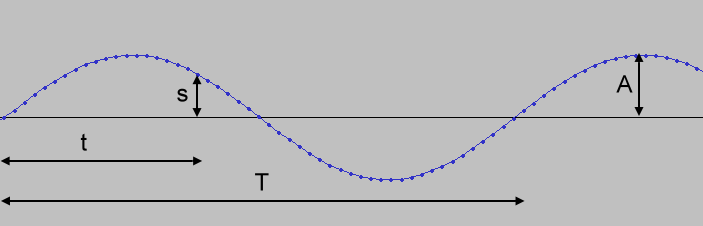Album

506室內 2020-11-04 21:43:575樓室外 2020-11-04 21:43:43# Sine wave generator

>>Sine wave ...

## Wave file format

A wave file consists of a 44 bytes header and sound wave samples.

data chunk size = number of sample frames * 4 (4 bytes for stereo and 16 bit samples)
file size = 44 + data chunk size
numbers are stored with least significant byte first (little-endian)
- value=file size-8=data chunk size+36
- number of channels, stereo=2
- sampling rate
- byte rate=sampling rate * NumChannels * BitsPerSample/8
- bits per sample, value 16 for 16 bits
- data chunk size
- the sound samples (16 bit signed integer, 2 bytes for the left followed by 2 bytes for the right)

ref: bit rate for 44100Hz 16bit stereo = 1411200bps

 Left (Hz): Right (Hz): Duration (max 8s):A=amplitude
f=frequency of the sine wave
T=period=1/f
R=sampling rate
t=time=i*1/R (i=loop counter)
s=sample=A*sin(2*pi*t/T)
=A*sin(2*pi*f*t)
=A*sin(2*pi*f*i/R)

Unlike many other programming languages, JavaScript numbers are always stored as double precision floating point numbers. 16-bit wave samples are stored as 2's-complement signed integers, ranging from -32768 to 32767 (little-endian). Therefore, negative JavaScript numbers must be converted to 2's complement format before converting to base64 characters.
if (s<0) s=s+65536;

ref: WAVE PCM sound file formatAlbum

506室內 2020-11-04 21:43:575樓室外 2020-11-04 21:43:43⇧